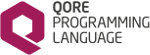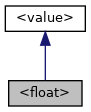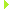Qore Programming Language Reference Manual  1.7.0
<float> Class Reference

Methods in this pseudo-class can be executed on floating-point values. More...

Inheritance diagram for Qore::<float>:[legend]

## Public Member Methods

float abs ()
Returns the absolute value of the number. More...

string format (string fmt)
Returns a string of a formatted number according to a format string. More...

string format (int prec=2, string decimal_sep=".", string thousands_sep="")
Returns a formatted string of the floating-point number according to format arguments. More...

bool infp ()
Returns True if the number is infinity (+ or -) More...

bool intp ()
Returns True because float values can be converted to integers. More...

bool nanp ()
Returns True if the number is NaN (not a number) More...

int sign ()
Returns -1 if the number is negative, 0 if it is zero, or 1 if it is positive. More...

bool strp ()
Returns True because float values can be converted to strings. More...

string toBase (int base=10)
Converts the number to a different base (and returns it as a string). More...

int typeCode ()
Returns Qore::NT_FLOAT. More...

bool val ()
Returns True if the float is non-zero, False if zero. More...Public Member Methods inherited from <value>
bool callp ()
Returns False; this method is reimplemented in other types and will return True if the given expression is a callable value (ie closures or call references) More...

bool complexType ()
returns True if the value has a complex type, False if not More...

bool empty ()
Returns True; this method will be reimplemented in container types where it may return False. More...

string fullType (*bool with_namespaces)
returns the full type name which differs from the simple type name in case of complex types and objects More...

bool intp ()
Returns False; this method is reimplemented in other types and will return True if the given expression can be converted to an integer. More...

AbstractIterator iterator ()
Returns an iterator object for the value; the default iterator object returned is SingleValueIterator. More...

int lsize ()
Returns 1; the return value of this method should give the list size of the value, which is normally 1 for non-lists (except for NOTHING where the size will be 0) and the number of the elements in the list for lists; this method will be reimplemented in other types where it may return other values. More...

int size ()
Returns zero; this method will be reimplemented in container types where it may return a non-zero value. More...

bool sizep ()
Returns True if the type can return a non-zero size (True for containers including binary objects and strings, False for everything else) More...

bool strp ()
Returns False; this method is reimplemented in other types and will return True if the given expression can be converted to a string. More...

bool toBool ()
Returns the boolean representation of the value; the default is False. More...

float toFloat ()
Returns the floating-point representation of the value; the default is 0.0. More...

int toInt ()
Returns the integer representation of the value; the default is 0. More...

number toNumber ()
Returns the arbitrary-precision numeric representation of the value; the default is 0. More...

string toString ()
Returns the string representation of the value; the default is an empty string. More...

string type ()
Returns the string type for the value. More...

int typeCode ()
Returns the type code for the value. More...

bool val ()
Returns False; this method is reimplemented in other types and will return True if the given expression has a non-empty value. More...

## Detailed Description

Methods in this pseudo-class can be executed on floating-point values.

## ◆ abs()

 float ::abs ( )

Returns the absolute value of the number.

Code Flags:
CONSTANT
Example:
f = f.abs();
Returns
the absolute value of the number
Note
equivalent to abs(float)
Since
Qore 0.8.8

## ◆ format() [1/2]

 string ::format ( int prec = `2`, string decimal_sep = `"."`, string thousands_sep = `""` )

Returns a formatted string of the floating-point number according to format arguments.

Code Flags:
RET_VALUE_ONLY
Parameters
 prec the number of digits after the decimal point; a negative value means to round that number of digits before the decimal place, whereas the special constant SNF_AllDigits means to include all possible digits after the decimal point decimal_sep a single character that defines the decimal separator; an empty string means no decimal separator thousands_sep a single character that defines the thousands and further separators every three digits before the decimal point; an empty string means no thousands separator
Returns
a string representing the floating-point number according to the format arguments as described above
Example:
number n = -48392093894.2349n;
string nstr = n.format(3, ".", ","); # returns "-48.392.093.894,235"
Since
Qore 0.8.13

## ◆ format() [2/2]

 string ::format ( string fmt )

Returns a string of a formatted number according to a format string.

Code Flags:
CONSTANT
Parameters
 fmt the format string has the following format: `[`[]`]` where: thousands_separator and decimal_separator are single characters defining the thousands and decimal separator characters respectively, and decimals is one or more digits defining how may decimals should appear after the decimal point; a negative number means to round that number of digits before the decimal place
Returns
a string of a formatted number according to a format string; if the format string does not follow the given format, then the number without formatting is returned
Example:
float f = -48392093894.2349;
string nstr = f.format(".,3"); # returns "-48.392.093.894,235"
Note
equivalent to format_number(string, softfloat)
Since
Qore 0.8.6

## ◆ infp()

 bool ::infp ( )

Returns True if the number is infinity (+ or -)

Returns
True if the number is infinity (+ or -)
Code Flags:
CONSTANT
Example:
if (f.infp())
print("the operation resulted in infinity\n");
nothing print(...)
Outputs a string to standard output with no formatting.
Since
Qore 0.8.12

## ◆ intp()

 bool ::intp ( )

Returns True because float values can be converted to integers.

Returns
True because float values can be converted to integers
Code Flags:
CONSTANT
Example:
if (n.intp())
printf("%y: can be converted to an integer: %d\n", n, int(n));
string printf(string fmt,...)
Outputs the string passed to standard output, using the first argument as a format string; does not e...
int int(string str, int base=10)
Converts the string to an integer value with respect to the base.

## ◆ nanp()

 bool ::nanp ( )

Returns True if the number is NaN (not a number)

Returns
True if the number is NaN (not a number)
Code Flags:
CONSTANT
Example:
if (f.nanp())
print("the operation resulted in NaN\n");
Since
Qore 0.8.12

## ◆ sign()

 int ::sign ( )

Returns -1 if the number is negative, 0 if it is zero, or 1 if it is positive.

Returns
-1 if the number is negative, 0 if it is zero, or 1 if it is positive
Code Flags:
CONSTANT
Example:
printf("sign: %d\n", f.sign());
Since
Qore 0.8.6

## ◆ strp()

 bool ::strp ( )

Returns True because float values can be converted to strings.

Returns
True because float values can be converted to strings
Code Flags:
CONSTANT
Example:
if (n.strp())
printf("%y: can be converted to a string: '%s'\n", n, string(n));
string string(softstring str, *string enc)
Converts the argument to a string.

## ◆ toBase()

 string ::toBase ( int base = `10` )

Converts the number to a different base (and returns it as a string).

Code Flags:
RET_VALUE_ONLY
Parameters
 base the base to convert the number to; this value must be 2 - 36 inclusive or an `INVALID-BASE` exception will be thrown
Returns
the converted value as a string
Example:
(15.5).toBase(20)); // returns "F.A"
(-13.8).toBase(20)); // returns "-D.G"
(21.48).toBase(15)); // returns "16.73"
Exceptions
 INVALID-BASE the base is invalid; must be 2 - 36 inclusive
<int>::toBase(int base)
<number>::toBase(int base)
Since
Qore 0.8.13

## ◆ typeCode()

 int ::typeCode ( )

Returns Qore::NT_FLOAT.

Returns
Qore::NT_FLOAT
Code Flags:
CONSTANT
Example:
switch (f.typeCode()) {
case NT_FLOAT:
printf("%y: is a float\n", f);
break;
}

## ◆ val()

 bool ::val ( )

Returns True if the float is non-zero, False if zero.

Returns
True if the float is non-zero, False if zero
Code Flags:
CONSTANT
Example:
bool b = f.val();